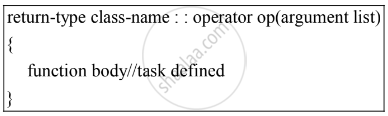# What is Operator Function? Describe the Syntax of an Operator Function. - Computer Science 1

Short Note

What is Operator Function? Describe the syntax of an operator Function.

#### Solution

(1) To define an additional task to an operator, it specify what it means in relation to the class to which the operator is applied. This is done with the help of a special function, called operator function, which describes the task.
(2) In short, a function which defines additional task to an operator or which gives a special meaning to an operator is called the operator function.
(3) The general form of operator function is,Where return type is the of value returned by the specified operation and op is the operator being overloaded.
The op is preceded by the keyword operator. Operator op is the function name.
(4) Operator functions must be either member functions (non-static) or friend functions.

Concept: C++ Programming
Is there an error in this question or solution?